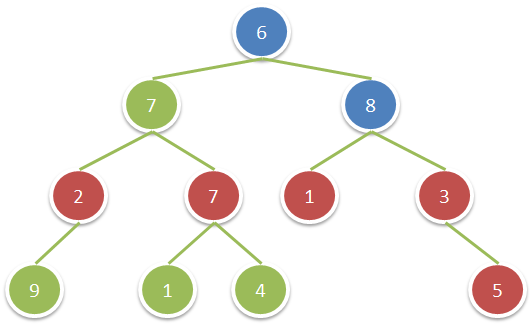## 1315. Sum of Nodes with Even-Valued Grandparent

Given a binary tree, return the sum of values of nodes with even-valued grandparent.  (A grandparent of a node is the parent of its parent, if it exists.)

If there are no nodes with an even-valued grandparent, return `0`.

Example 1:```Input: root = [6,7,8,2,7,1,3,9,null,1,4,null,null,null,5]
Output: 18
Explanation: The red nodes are the nodes with even-value grandparent while the blue nodes are the even-value grandparents.
```

Constraints:

• The number of nodes in the tree is between `1` and `10^4`.
• The value of nodes is between `1` and `100`.

## Rust Solution

``````struct Solution;
use rustgym_util::*;

trait Preorder {
fn preorder(&self, parent: bool, grand_parent: bool, sum: &mut i32);
}

impl Preorder for TreeLink {
fn preorder(&self, parent: bool, grand_parent: bool, sum: &mut i32) {
if let Some(node) = self {
let val = node.borrow().val;
let left = &node.borrow().left;
let right = &node.borrow().right;
if grand_parent {
*sum += val;
}
left.preorder(val % 2 == 0, parent, sum);
right.preorder(val % 2 == 0, parent, sum);
}
}
}

impl Solution {
fn sum_even_grandparent(root: TreeLink) -> i32 {
let mut res = 0;
root.preorder(false, false, &mut res);
res
}
}

#[test]
fn test() {
let root = tree!(
6,
tree!(7, tree!(2, tree!(9), None), tree!(7, tree!(1), tree!(4))),
tree!(8, tree!(1), tree!(3, None, tree!(5)))
);
let res = 18;
assert_eq!(Solution::sum_even_grandparent(root), res);
}
``````

Having problems with this solution? Click here to submit an issue on github.